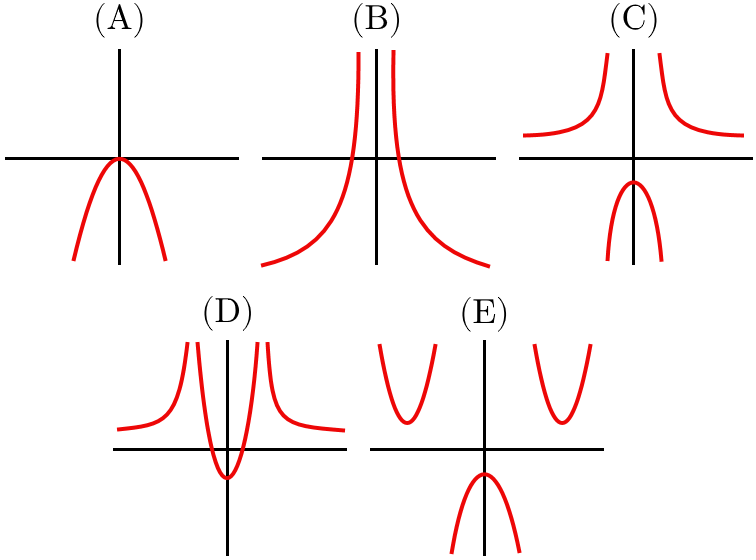# Sketching Curves 2

Calculus Level 2The graph of the function $y=\dfrac{x^2+1}{x^2-1}$ is sketched in which set of axes?

×